# What is the importance of the contact force

## friction

In the following article you will find explanations, tasks and videos on the topic of friction. We deal with the various conditions as well as the topic of rope friction:

Technical mechanics learning booklet with comprehensible explanations with matching StudyHelp-TV learning videos

19,99€

### definition

Friction is a resistance in the contact surfaces of two bodies, which prevents or at least impairs mutual movement by sliding, rolling or rolling.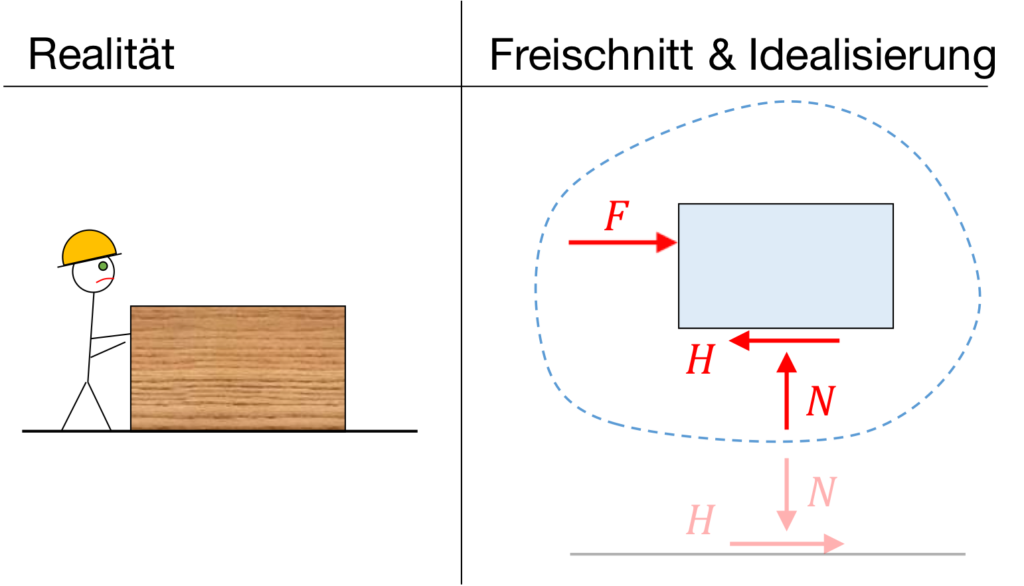If in "reality" a construction worker tries to push a box away, the friction force \$ H \$ acts against it, as you can see in the free cut. In addition, there is a normal force \$ N \$.

Whether the box slips depends on the nature of the box, the subsurface and the applied force \$ F \$.

We differentiate between 4 different states of friction (see Rolf Mahnken, Textbook of Technical Mechanics - Statics, Springer Verlag, 1st edition, 2012):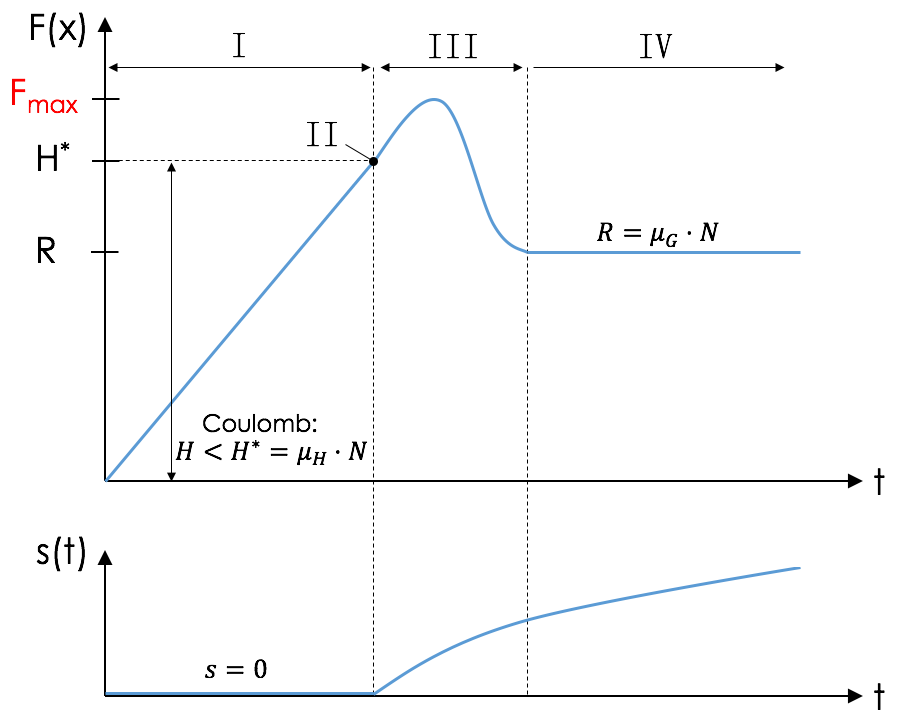I - state of static friction

The state of static friction describes the retirement, in which all forces acting on the body are in equilibrium.

II - limit state

In the limit state the frictional force \$ H \$ reaches the limit static friction force \$ H ^ * \$.

III - state of acceleration

In this state the body is accelerated for a short time.

IV - state of sliding friction

Here the body is in equilibrium in uniform motion at constant speed. Instead of the frictional force \$ H \$, the contact force \$ R ^ * \$ acts.

Important for us:

State I and II belong to Statics (this ebook)

State III and IV belong to dynamics!

For tasks on the topic of friction in statics, the task text must be read carefully! As soon as information such as “maximum”, “minimum”, “maximum” etc. is there, you are in state II and you have to work with Coulomb's law of static friction. The following applies:

\ begin {align *}
H ^ * = \ mu_H \ cdot N
\ end {align *}

with \$ \ mu_H \$ as the coefficient of static friction, which depends on the

• Material pairing
• Geometric, physical surface
• temperature

and \$ N \$ as normal force.

### example

A box with the mass \$ m \$ lies on an inclined plane, see illustration.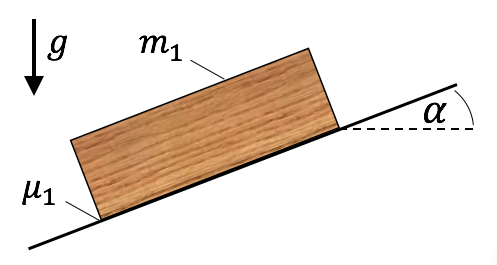a) Determine the reaction forces in the contact area.

b) How big must \$ \ alpha ^ * \$ be so that no slipping occurs? How big are the reaction forces then?

Known: \$ \ alpha = 30 ^ o, \ m = 10 \ \ textrm {kg}, \ mu_1 = 0.6, \ g = 10 \ \ textrm {m / s} ^ 2 \$

Solution steps for a)

1. Draw free cut: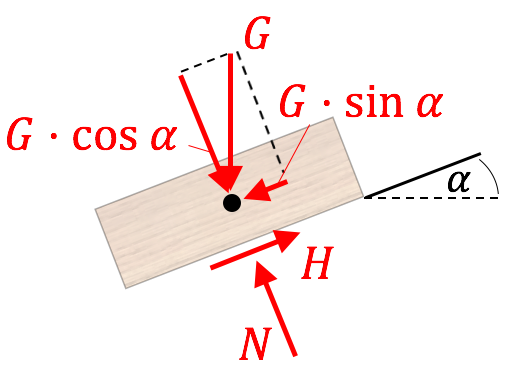2. Calculation of the reaction forces:

The unknowns are \$ N \$ and \$ H \$. For this we simply set up two equilibrium conditions.

\ begin {align *}
\ searrow: \ G \ cdot \ cos \ alpha -N = 0 \ & \ Leftrightarrow \ N = G \ cdot \ cos \ alpha = 86.6 \ \ textrm {N} \
\ nearrow: \ H - G \ cdot \ sin \ alpha = 0 \ & \ Leftrightarrow \ H = G \ cdot \ sin \ alpha = 50 \ \ textrm {N}
\ end {align *}

According to the law of static friction (Coulumb), the following applies to the maximum static friction force:

\ begin {align *}
H ^ * = \ mu_1 \ times N = 0.6 \ times 86.6 \ \ textrm {N} = 51.96 \ \ textrm {N}> 50 \ \ textrm {N} = H
\ end {align *}

Since the limit state \$ H ^ * \$ is greater than the static friction \$ H \$ that occurs, the box remains at rest.

Solution steps for b)

We use the cutout from a) - but here we are in the limit state! This note is given in the text: “No slipping at the moment”. For this reason we now enter the unknowns \$ N ^ * \$, \$ H ^ * \$ and \$ \ alpha ^ * \$ in the cut-out.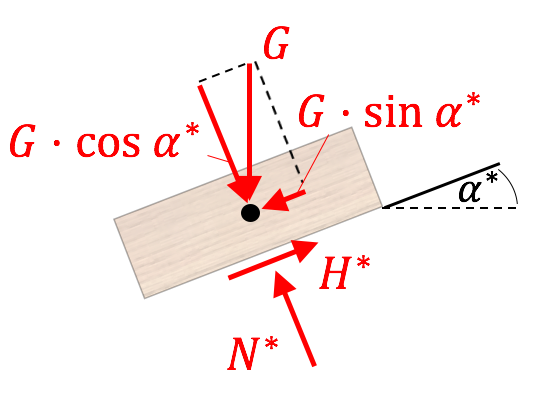Since we are in the limit state, we can use the law of static friction. We need that as an additional third equation to determine the three unknowns. To do this, we set the equilibrium conditions

\ begin {align *}
H ^ * = G \ cdot \ sin \ alpha ^ * \ quad \ textrm {and} \ quad N ^ * = G \ cdot \ cos \ alpha ^ *
\ end {align *}

into the law of static friction \$ H ^ * = \ mu_1 \ cdot N ^ * \$:

\ begin {align *}
G \ cdot \ sin \ alpha ^ * = \ mu_1 \ cdot G \ cdot \ cos \ alpha ^ * \ \ Leftrightarrow \ \ frac {\ sin \ alpha ^ *} {\ cos \ alpha ^ *} = \ mu_1 \ \ Rightarrow \ tan \ alpha ^ * = \ mu_1
\ end {align *}

From this it follows for the angle: \$ \ alpha ^ * = \ arctan \ mu_1 = 30.96 ^ o> \ alpha \$.

The reaction forces are:

\ begin {align *}
H ^ * = G \ cdot \ sin \ alpha ^ * = 51.44 \ \ textrm {N} \ quad \ textrm {and} \ quad N ^ * = G \ cdot \ cos \ alpha ^ * = 85.75 \ \ textrm {N}
\ end {align *}

Video simple example of friction

Friction - Technical Mechanics 1 - Statics

Technical mechanics learning booklet with comprehensible explanations with matching StudyHelp-TV learning videos

19,99€

### Rope friction

Rope friction occurs when a rope is laid over a curved surface (e.g. a cylinder) and is subjected to two forces at both ends.

In the limit state, we can establish the relationship between the rope forces using the rope friction formula according to Euler and Eytelwein.

It must first be clarified which side is the load side.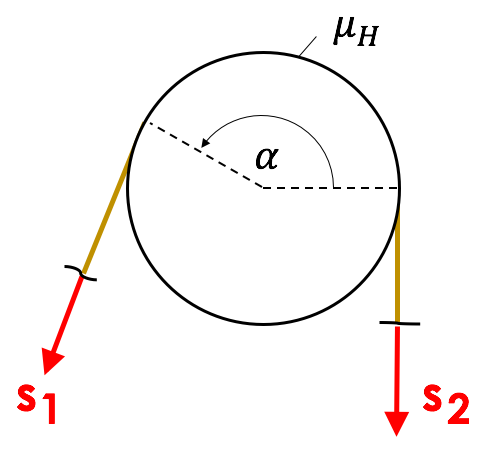If \$ s_2> s_1 \$, then the following applies:

\ begin {align *}
s_2 = s_1 \ cdot e ^ {\ mu \ cdot \ alpha}
\ end {align *}

with \$ \ alpha \$ as the wrap angle - this must be given in radians!

Note on converting angles to radians:

Multiply the angle in degrees by \$ \ frac {\ pi} {180 ^ o} \$, e.g. 360 ° in radians are \$ 360 \ cdot \ frac {\ pi} {180 ^ o} = 2 \ pi \$.

### Example of static and rope friction in combination

The following system is given. In addition to static friction on the box, rope friction also occurs in this task.

How heavy does the box with the mass \$ m_2 \$ have to be, so that the box with the mass \$ m_1 \$ does not just follow

a) above and

b) slips down?

Known: \$ m_1 = 50 \ \ textrm {kg}, \ m_2 = 0.5 m_1, \ \ alpha = 20 ^ o, \ \ beta = \ gamma = 30 ^ o, \$ \$ \ mu_1 = 0.6, \ \ mu_2 = \ mu_3 = 0.2, \ g = 10 \ \ textrm {m / s} ^ 2 \$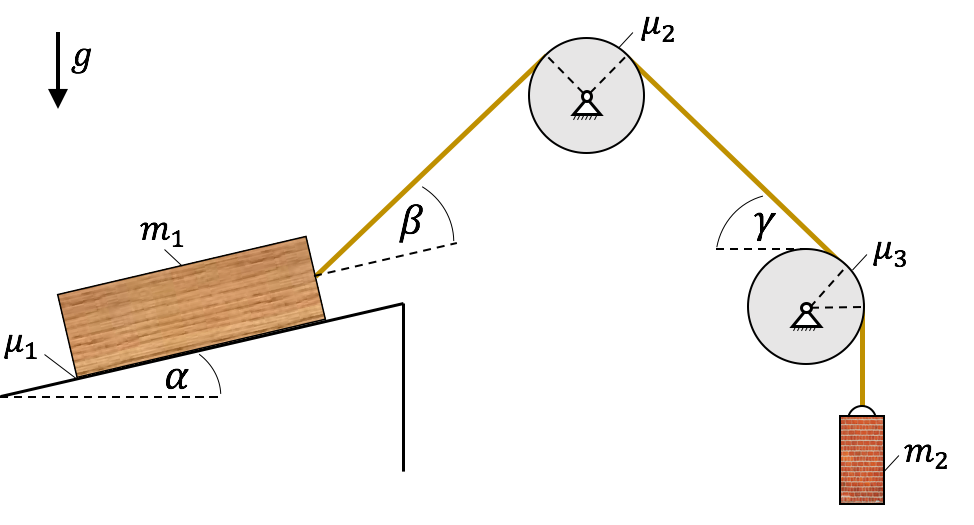Video - similar example of static friction and rope friction

Rope friction - friction - free cut - technical mechanics 1
Technical mechanics learning booklet with understandable explanations with matching StudyHelp TV learning videos

19,99€